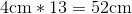## Example Questions

### Example Question #1 : Plane Geometry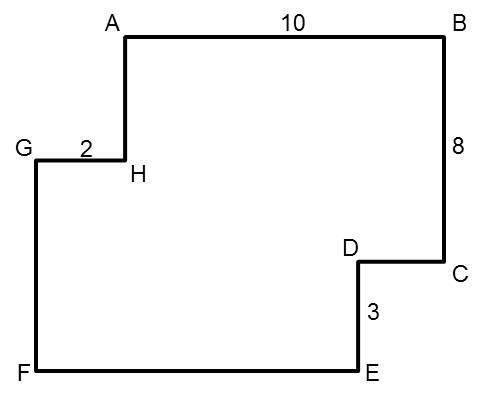All segments of the polygon meet at right angles (90 degrees). The length of segment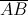is 10. The length of segment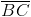is 8. The length of segment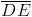is 3. The length of segment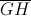is 2.

Find the perimeter of the polygon.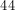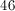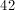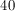Explanation:

The perimeter of the polygon is 46. Think of this polygon as a rectangle with two of its corners "flipped" inwards. This "flipping" changes the area of the rectangle, but not its perimeter; therefore, the top and bottom sides of the original rectangle would be 12 units long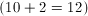. The left and right sides would be 11 units long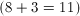. Adding all four sides, we find that the perimeter of the recangle (and therefore, of this polygon) is 46.

### Example Question #2 : How To Find The Perimeter Of A Polygon

In the figure below, each pair of intersecting line segments forms a right angle, and all the lengths are given in feet. What is the perimeter, in feet, of the figure?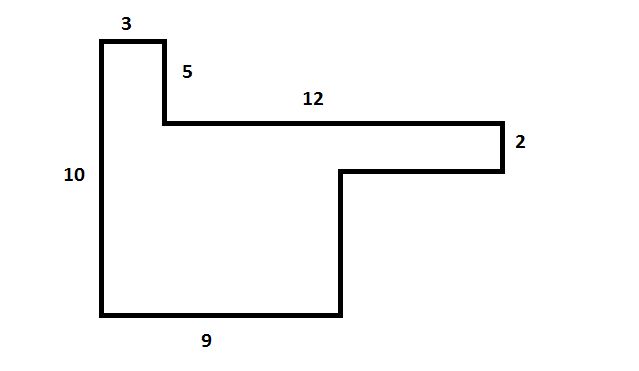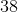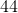Explanation:

Fill in the missing sides by thinking about the entire figure as a big rectangle. In the figure below, the large rectangle is outlined in blue and the missing numbers are supplied in red.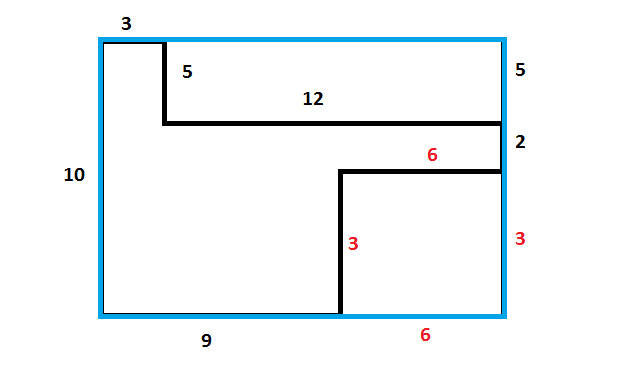### Example Question #3 : How To Find The Perimeter Of A Polygon

What is the perimeter of a regular,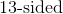polygon with a side length of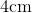?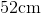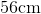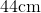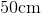To find the perimeter of an-sided polygon with a side length of, simply multiply the side length by the number of sides: